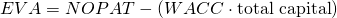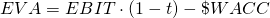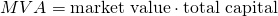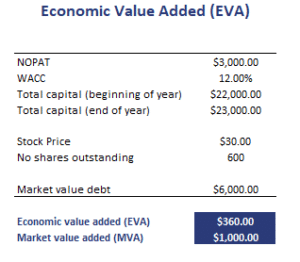Economic Value Added (EVA) analysis measures the value added for shareholders by management over a given year. It is not the same as residual income. Whereas residual income starts from net income after interest expense, EVA uses the net operating profit after tax (NOPAT). Still, both measures are used by analysts to measure economic income. Another related measure is market value added. EVA is related but not completely the same. Thus, we also discuss the Market Value Added (MVA) so we can distinguish between the two.

How to calculate economic value added? There are two formulas we can use to calculate EVA. The first economic value added formula equalswhere NOPAT is the net operating profit after tax, WACC is the after-tax weighted average cost of capital in decimal terms, and total capital is the net working capital plus net fixed assets. Alternatively, total capital can also be calculated as the book value of long-term debt plus the book value of equity.

A second way to calculate EVA is using Earnings Before Interest and Tax (EBIT)where t is the marginal tax rate and \$WACC is the dollar cost of capital.

The advantage of EVA is that it is a measure of economic income rather than accounting income. Thus, it better reflects the economic performance of the company.

Market value added (MVA) is similar to EVA. MVA measures the management’s added value since the company’s inception. It is calculated asBefore calculating NOPAT and total capital, the analyst may make any of the following adjustments:

• Treat operating leases as capital leases
• Capitalize and amortize R&D
• Add the LIFO reserve to invested capital and add change in LIFO reserve to NOPAT
• Add back charges on strategic investments
• Eliminate deferred taxes

Let’s finish with an economic value added calculation example. The table below applies the formula using data on all the variables discussed above. The spreadsheet is available for download below.## Summary

We discussed the EVA, a method that is commonly used to evaluate management’s added value in a given year.

### Return calculation

Want to have an implementation in Excel? Download the Excel file: EVA calculator